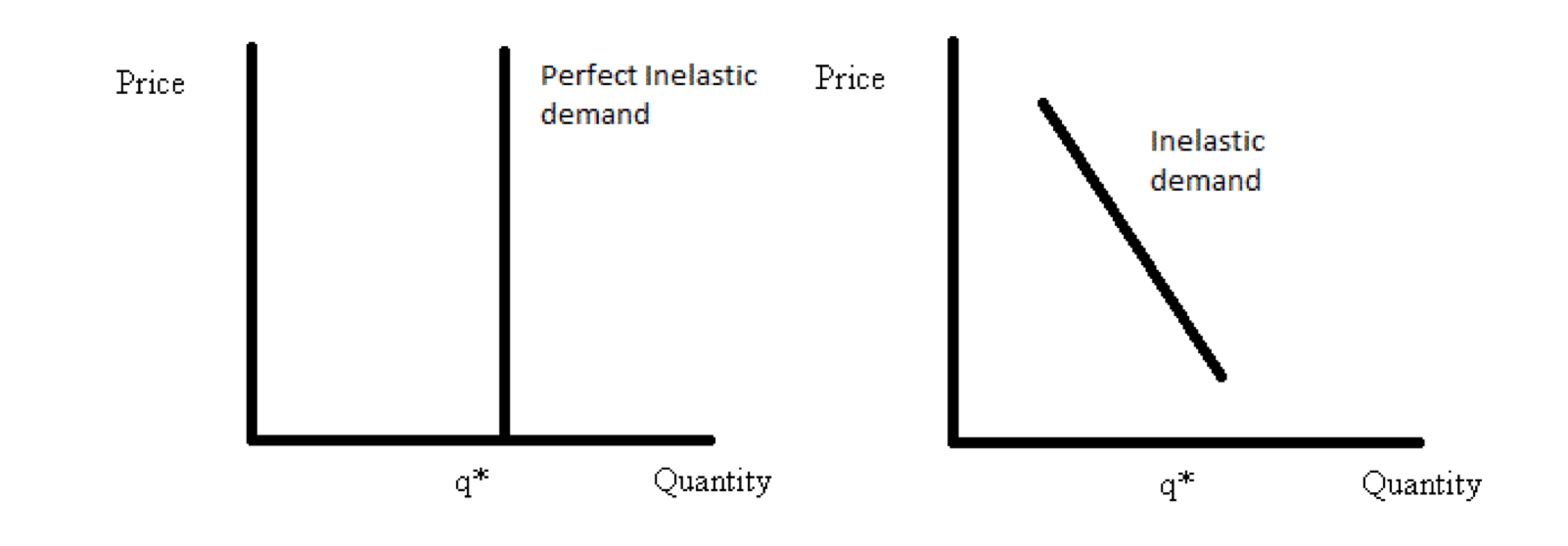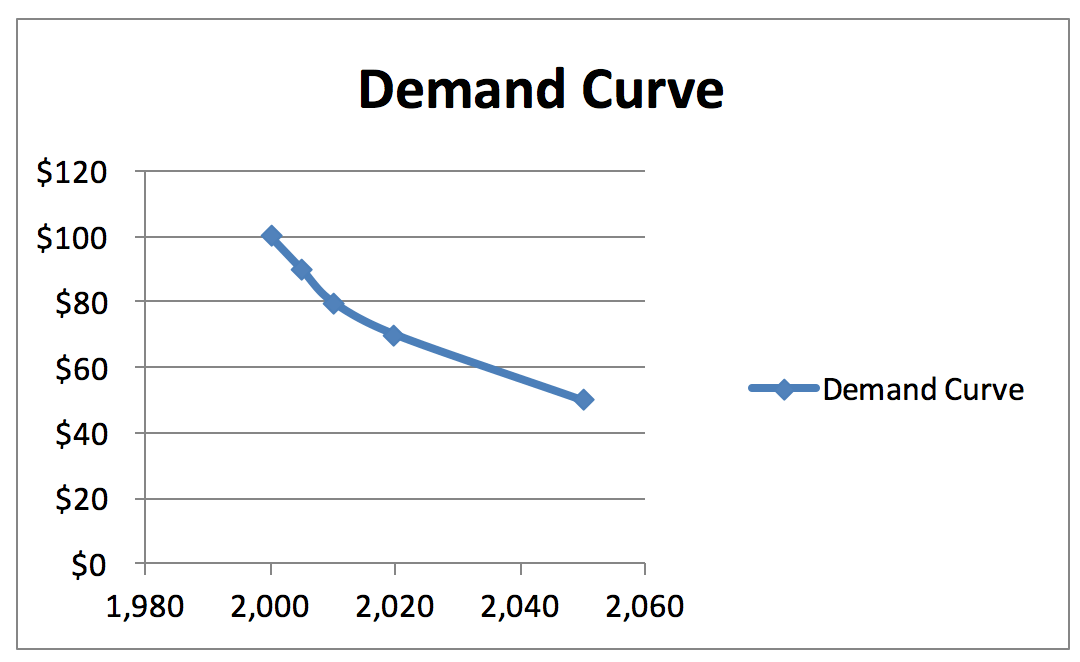# Inelastic Demand

Little or no change in demand alongside the change in prices

## What is Inelastic Demand?

Inelastic demand is when the buyer’s demand does not change as much as the price changes. When price increases by 20% and demand decreases by only 1%, demand is said to be inelastic.

This situation typically occurs with everyday household products and services. When the price increases, people will still purchase roughly the same amount of the good or service as they did prior to the increase because their needs stay the same. A similar situation exists when there is a decrease in price – demand will not increase substantially because consumers only have a limited need for the product(s).

### Elasticity Formula:

#### Elasticity = Percentage Change in Demand / Percentage Change in Price

For example, look at the demand and price table below:

PriceQuantity of demandDemand situation
\$1002,000I
\$902,005II
\$802,010III
\$702,020IV
\$502,050V

### Calculating Change in Demand Situation I to II

##### Elasticity = -0.0949

This number shows that a price decrease of 1% will increase demand by 0.0949%.

### Demand Curve

There are two types of demand curves:

1.  Perfectly inelastic demand

2.  Inelastic demand

An example of the two types of curves are shown below:Note: Perfectly inelastic demand is when a change in prices does not change the quantity of demand at all.

### Drawing the Demand Curve Using Example DataUsing data from the example calculation, a demand curve is drawn by placing the price on the Y-axis and demand on the X-axis. The line drawn from the example data results in an inelastic demand curve.

### Types of Elasticity of Demand

There are five types of elasticity of demand:

1.   Perfectly elastic demand

2.   Perfectly inelastic demand

3.   Unitary demand

4.   Elastic demand

5.   Inelastic demand

Perfectly inelastic demand means that prices or quantities are fixed and are not affected by the other variable. Unitary demand occurs when a change in price causes a perfectly proportionate change in quantity demanded.